Multiple lines of symmetry

Chapter 12 Class 7 Symmetry
Concept wise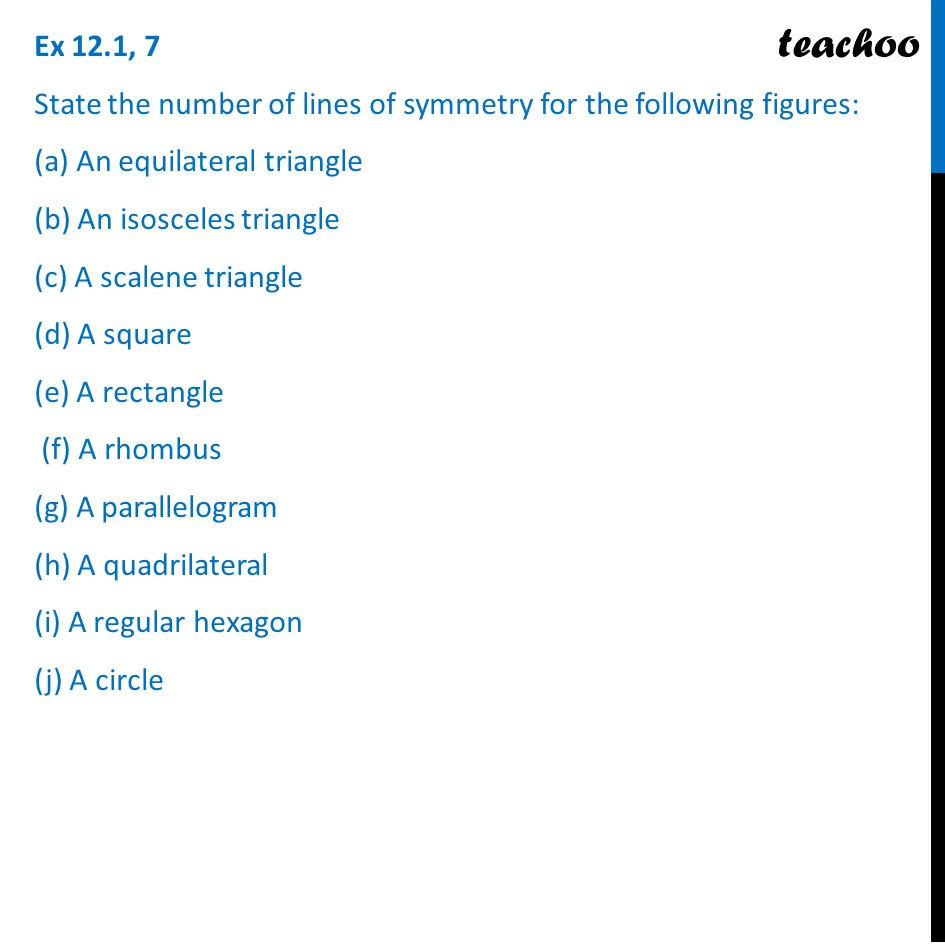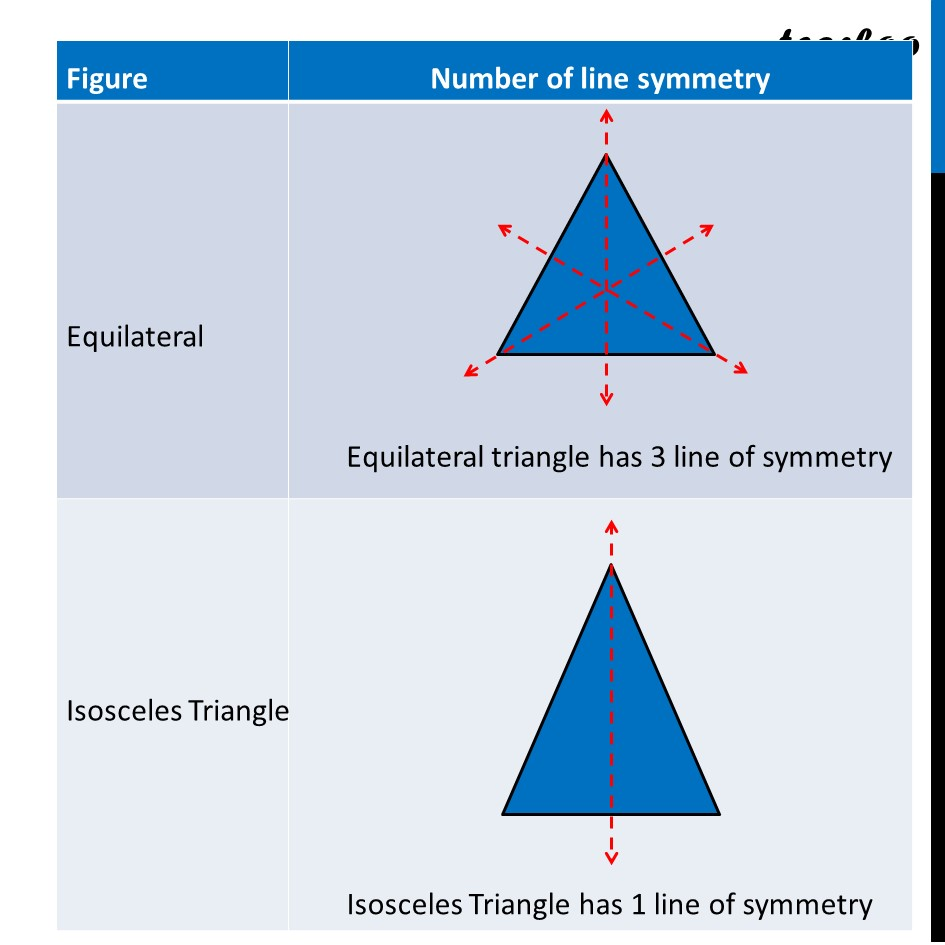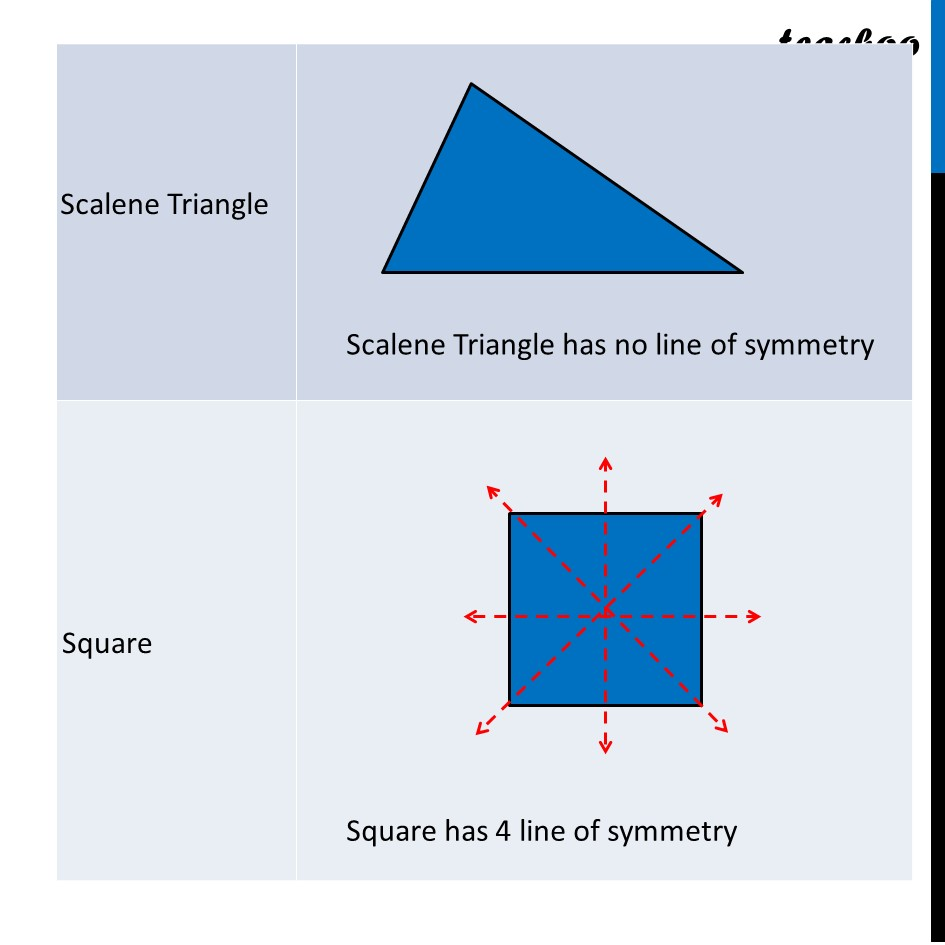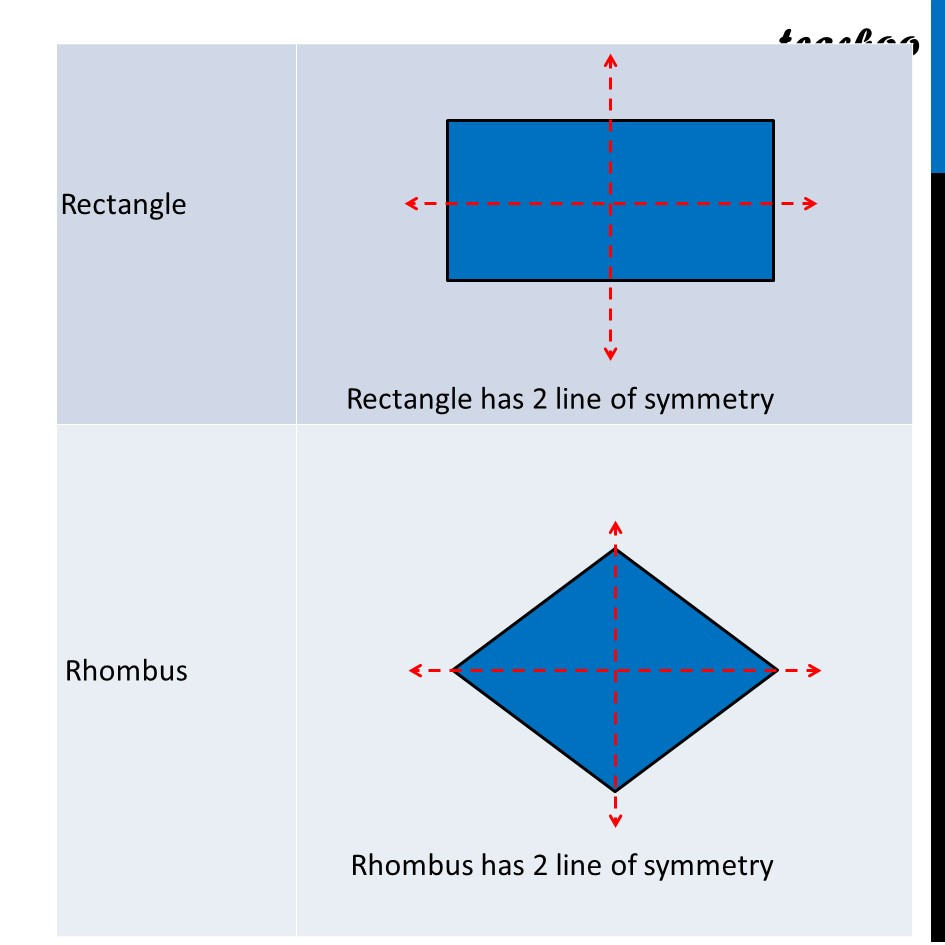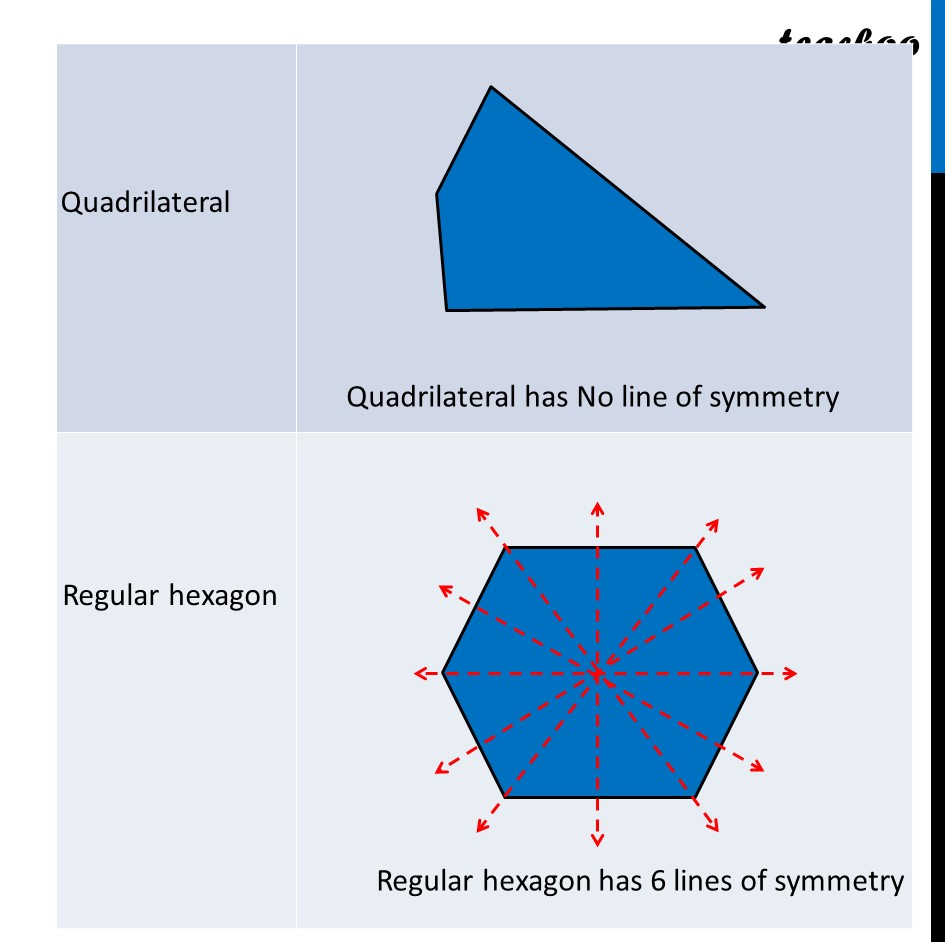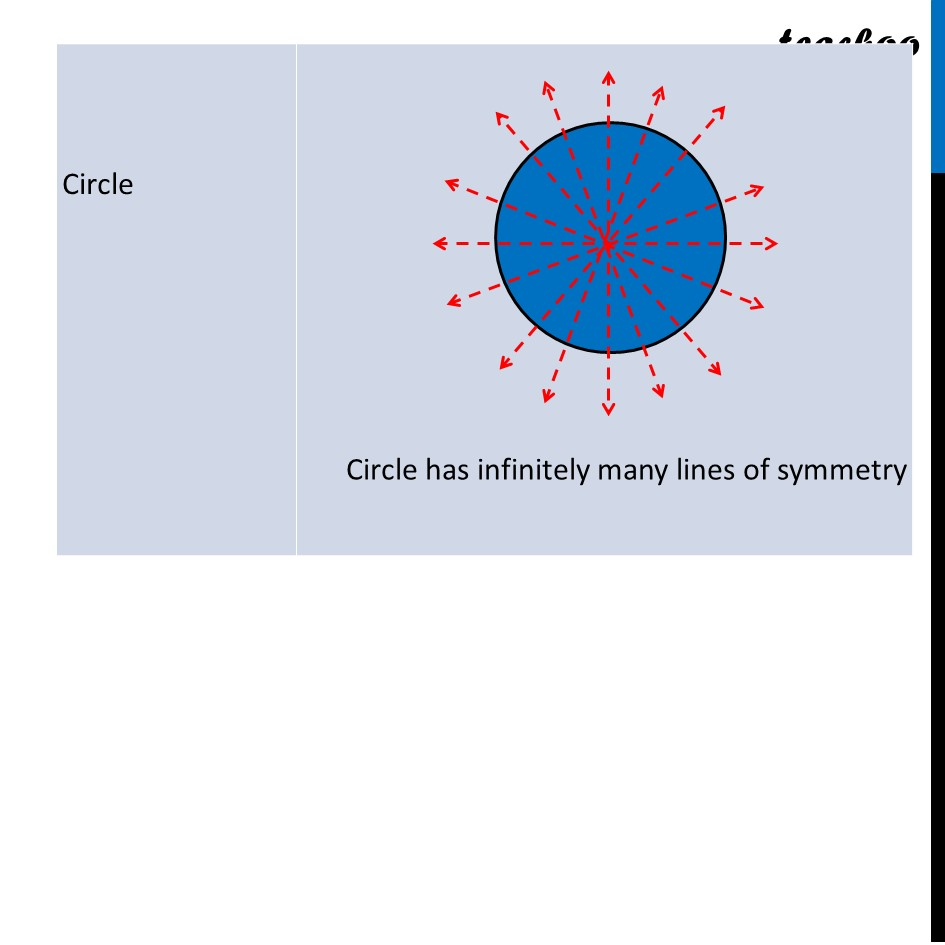Learn in your speed, with individual attention - Teachoo Maths 1-on-1 Class

### Transcript

Ex 12.1, 7 State the number of lines of symmetry for the following figures: (a) An equilateral triangle (b) An isosceles triangle (c) A scalene triangle (d) A square (e) A rectangle (f) A rhombus (g) A parallelogram (h) A quadrilateral (i) A regular hexagon (j) A circle